Publicité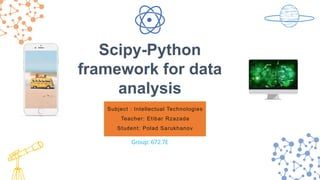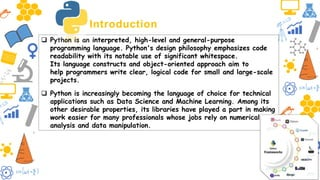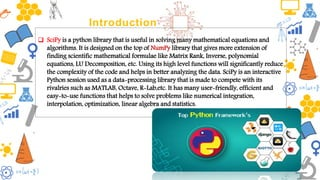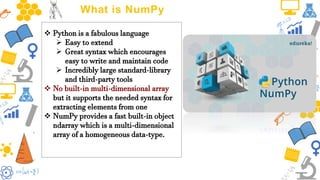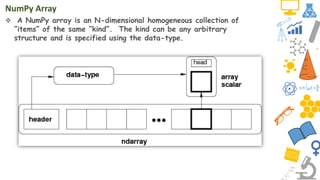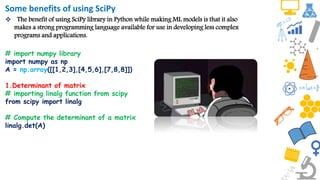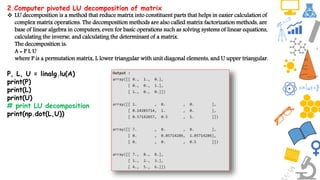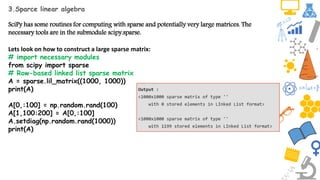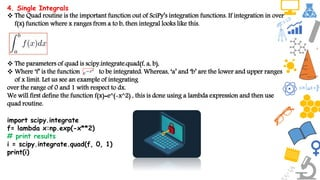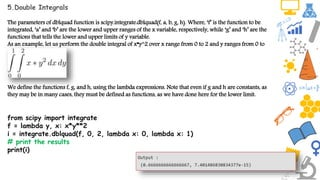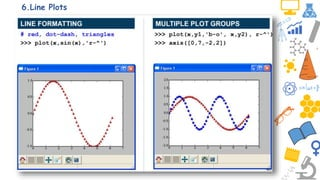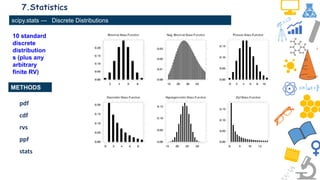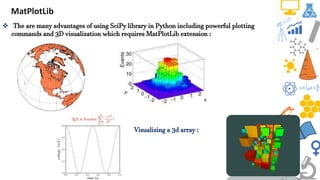1 sur 15
Publicité

### Intellectual technologies

1. Subject : Intellectual Technologies Teacher: Etibar Rzazada Student: Polad Sarukhanov Scipy-Python framework for data analysis Group: 672.7E
2. Introduction  Python is an interpreted, high-level and general-purpose programming language. Python's design philosophy emphasizes code readability with its notable use of significant whitespace. Its language constructs and object-oriented approach aim to help programmers write clear, logical code for small and large-scale projects.  Python is increasingly becoming the language of choice for technical applications such as Data Science and Machine Learning. Among its other desirable properties, its libraries have played a part in making work easier for many professionals whose jobs rely on numerical analysis and data manipulation.
3. Introduction  SciPy is a python library that is useful in solving many mathematical equations and algorithms. It is designed on the top of NumPy library that gives more extension of finding scientific mathematical formulae like Matrix Rank, Inverse, polynomial equations, LU Decomposition, etc. Using its high level functions will significantly reduce the complexity of the code and helps in better analyzing the data. SciPy is an interactive Python session used as a data-processing library that is made to compete with its rivalries such as MATLAB, Octave, R-Lab,etc. It has many user-friendly, efficient and easy-to-use functions that helps to solve problems like numerical integration, interpolation, optimization, linear algebra and statistics.
4. SciPy Sub-packages  SciPy is organized into sub-packages covering different scientific computing domains. These are summarized in the following table −
5. What is NumPy  Python is a fabulous language  Easy to extend  Great syntax which encourages easy to write and maintain code  Incredibly large standard-library and third-party tools  No built-in multi-dimensional array but it supports the needed syntax for extracting elements from one  NumPy provides a fast built-in object ndarray which is a multi-dimensional array of a homogeneous data-type.
6. NumPy Array  A NumPy array is an N-dimensional homogeneous collection of “items” of the same “kind”. The kind can be any arbitrary structure and is specified using the data-type.
7. Some benefits of using SciPy  The benefit of using SciPy library in Python while making ML models is that it also makes a strong programming language available for use in developing less complex programs and applications. # import numpy library import numpy as np A = np.array([[1,2,3],[4,5,6],[7,8,8]]) 1.Determinant of matrix # importing linalg function from scipy from scipy import linalg # Compute the determinant of a matrix linalg.det(A)
8. 2.Computer pivoted LU decomposition of matrix  LU decomposition is a method that reduce matrix into constituent parts that helps in easier calculation of complex matrix operations. The decomposition methods are also called matrix factorization methods, are base of linear algebra in computers, even for basic operations such as solving systems of linear equations, calculating the inverse, and calculating the determinant of a matrix. The decomposition is: A = P L U where P is a permutation matrix, L lower triangular with unit diagonal elements, and U upper triangular. P, L, U = linalg.lu(A) print(P) print(L) print(U) # print LU decomposition print(np.dot(L,U))
9. 3.Sparce linear algebra SciPy has some routines for computing with sparse and potentially very large matrices. The necessary tools are in the submodule scipy.sparse. Lets look on how to construct a large sparse matrix: # import necessary modules from scipy import sparse # Row-based linked list sparse matrix A = sparse.lil_matrix((1000, 1000)) print(A) A[0,:100] = np.random.rand(100) A[1,100:200] = A[0,:100] A.setdiag(np.random.rand(1000)) print(A)
10. 4. Single Integrals  The Quad routine is the important function out of SciPy’s integration functions. If integration in over f(x) function where x ranges from a to b, then integral looks like this.  The parameters of quad is scipy.integrate.quad(f, a, b),  Where ‘f’ is the function to be integrated. Whereas, ‘a’ and ‘b’ are the lower and upper ranges of x limit. Let us see an example of integrating over the range of 0 and 1 with respect to dx. We will first define the function f(x)=e^(-x^2) , this is done using a lambda expression and then use quad routine. import scipy.integrate f= lambda x:np.exp(-x**2) # print results i = scipy.integrate.quad(f, 0, 1) print(i)
11. 5.Double Integrals The parameters of dblquad function is scipy.integrate.dblquad(f, a, b, g, h). Where, ‘f’ is the function to be integrated, ‘a’ and ‘b’ are the lower and upper ranges of the x variable, respectively, while ‘g’ and ‘h’ are the functions that tells the lower and upper limits of y variable. As an example, let us perform the double integral of x*y^2 over x range from 0 to 2 and y ranges from 0 to We define the functions f, g, and h, using the lambda expressions. Note that even if g and h are constants, as they may be in many cases, they must be defined as functions, as we have done here for the lower limit. from scipy import integrate f = lambda y, x: x*y**2 i = integrate.dblquad(f, 0, 2, lambda x: 0, lambda x: 1) # print the results print(i)
12. 6.Line Plots
13. 7.Statistics 10 standard discrete distribution s (plus any arbitrary finite RV) METHODS scipy.stats --- Discrete Distributions pdf cdf rvs ppf stats
14. MatPlotLib  The are many advantages of using SciPy library in Python including powerful plotting commands and 3D visualization which requires MatPlotLib extension : Visualizing a 3d array :
15. THANKS
Publicité